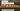# Array Constants

February 28, 2018

An Array Constant is one of those Excel features that goes virtually unnoticed. If you have ever evaluated a formula with `F9` (a handy shortcut) you may have noticed that an Excel range like `A1:A3` becomes `{1;2;3}`. That syntax of `{1;2;3}` is an array constant and it actually has some interesting uses if you get a little creative. In a previous post, we talked about Array Formulas for Beginners. This post builds on top of that and digs into what array constants are and how you can use them in your spreadsheets. Enjoy!

## What is an Array Constant?

In Excel, an array constant is a way to write an array of literal data in your Excel formulas.

Think of it this way, a range of `A1:A3` is actually an array of data. However, the data within that range can change and therefore is not considered “constant.” In an array constant, you specify the data, by hand, inside your Excel formula. This way, no matter where you copy your formula to or how the worksheet changes, the data in that array never changes, making it “constant.”

Let’s illustrate this point with an example. Let’s say you had this data:

If you made a `SUM` formula to add these up, it would look like:

``=SUM(A1:A3)``

If you went up to the formula bar, highlighted `A1:A3` and hit `F9`, the range would evaluate to `{1;2;3}`, which is an array constant.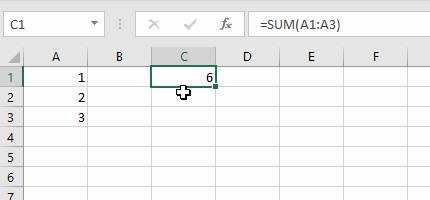In this case, the array constant of `{1;2;3}` is passed to the `SUM()` function and it adds up a total of `6`.

Here, we are creating an array constant manually and the `SUM()` function works as expected: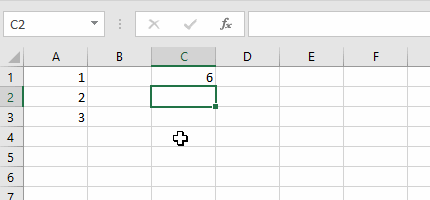As you can see, we are using an array constant for the formula in `C2`.## Array Constant Syntax

Now that we have some familiarity with array constants, let’s go over the syntax.

### Horizontal Array Constants

``={1,2,3}``

Notice the use of the comma to separate out the list of items. This array constant is synonymous to the range `A1:C1` where `A1=1`, `B1=2`, and `C1=3`.

To illustrate this further, select cells `A1:C1` and type that formula in the formula bar, then hit `Ctrl+Shift+Enter`: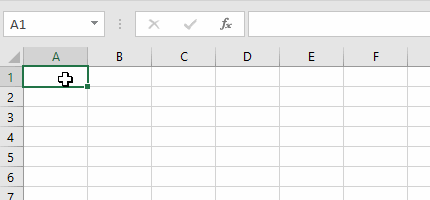As you can see, it placed each item in the array constant across the cells. It’s important to note that the amount of cells matched the amount of elements we have in the array constant. If we select too many cells, we will get `#N/A` errors.

Also, notice that we had to type in the curly braces manually for the array constant, and when we made it into an array formula, the extra curly braces were added to the entire formula. Remember that we must type in the curly braces manually when entering an array constant.

### Vertical Array Constants

``={1;2;3}``

Here, we use a semicolon to separate the different array items. Each semicolon is mean to represent a new row of data.

Select cells `A1:A3` and type in the above formula (along with the curly braces), then hit `Ctrl+Shift+Enter` to make it an array formula: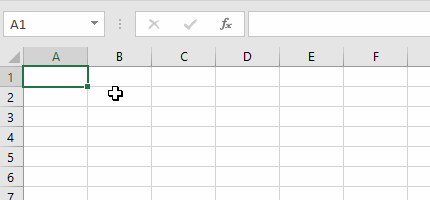### Two Dimensional Array Constants

Combining what we know about horizontal and vertical array constants, we can make a two dimensional array constant.

Select cells `A1:B2`, then type the following into the formula bar:

``={1,2;3,4}``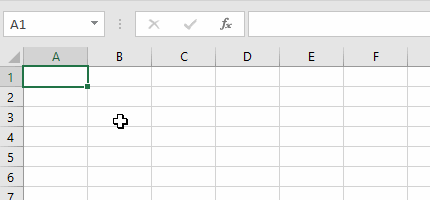The first row of data has two elements in it: `1` and `2`. The second row of data also has two elements in it: `3` and `4`. Each row is separated by a semicolon and each column of data is separated by a comma.

The distinction between commas and semicolons in array constants is important. If you mix any of these up, you can end up with some gotchas on your hands and your data may not look right.

What if you want to re-use your array constant? It can get annoying to repeat the same array constant over and over again. Luckily, we can place the array constant as a named range.

Let’s say we wanted to store the short names of the days of the week (Mon, Tue, etc.), we can do that by going to the Formulas tab and click Define Name like so: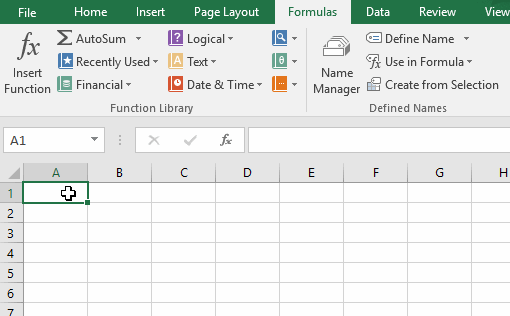There are some nice benefits to using this method:

• For short lists like this, you don’t have to create an entire worksheet to hold all of your lists.
• Having the list in a named range can shorten your formula length considerably.
• What’s nice about not having the named range data in a worksheet is that no changes to any worksheet will cause errors in your named range. For example, there have been times where a column gets deleted and your named range is left with #REF! errors. Not the case here!

Wow, you read the whole article! You know, people who make it this far are true learners. And clearly, you value learning. Would you like to learn more about Excel? Please consider supporting me by buying me a coffee (it takes a lot of coffee to write these articles!).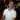Written by Joseph who loves teaching about Excel.